Submit a blog to KDnuggets -- Top Blogs Win A Reward

Topics: AI | Data Science | Data Visualization | Deep Learning | Machine Learning | NLP | Python | R | Statistics

KDnuggets Home » News » 2016 » Aug » Tutorials, Overviews » The Gentlest Introduction to Tensorflow – Part 1 ( 16:n31 )

# The Gentlest Introduction to Tensorflow – Part 1

In this series of articles, we present the gentlest introduction to Tensorflow that starts off by showing how to do linear regression for a single feature problem, and expand from there.

### Step 2: Create the Model in TF

Linear Model in TF

The 2 basic TF components are:

Placeholder: Represent an entry point for us to feed actual data values into the model when performing gradient descent, i.e., the house sizes (x), and the house prices (y_).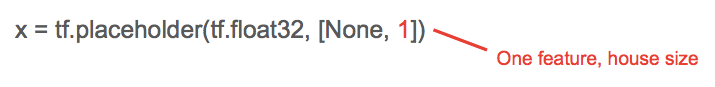Variable: Represent a variable that we are trying to find ‘good’ values that minimizes the cost function, e.g., W, and b.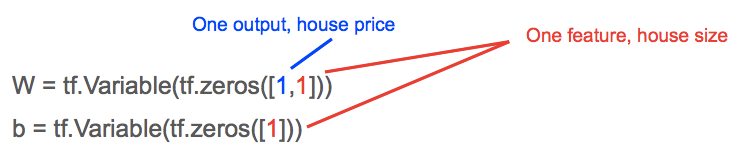The linear model (y = W.x + b) in TF then becomes: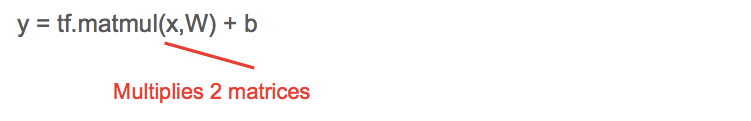Cost Function in TF

Similarly to feed actual house prices (y_) of the data points into the model, we create a placeholder.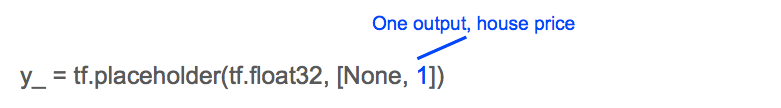Our cost function of least-min squared becomes: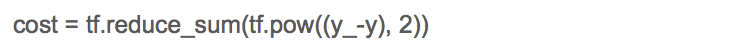Data

Since we do not have actual data points for house price (y_), house size (x), we generate them.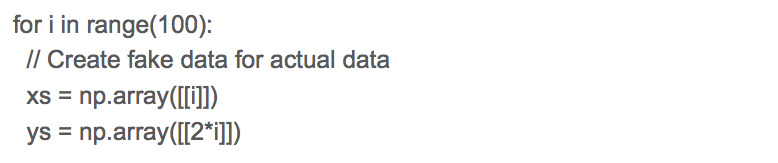We set the house price (ys) to always be 2 times the house size (xs) for simplicity.

With the linear model, cost function, and data, we can start performing gradient descent to minimize the cost function, to obtain the ‘good’ values for W, b.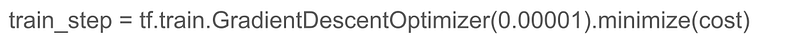The 0.00001 is the size of the ‘step’ we take in the direction of steepest gradient each time perform a training step; this is also called learning rate.

### Step 3: Train the Model

Training involves performing gradient descent a pre-determined number of times or until the cost is below a pre-determined threshold.

TF Quirks

All variables needs to be initialize at the start of training otherwise they may hold remnant values from previous execution.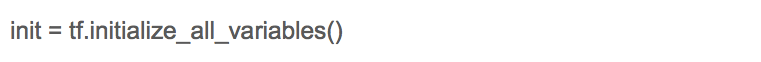TF Session

Although TF is a python library, and python is an interpreted language, TF operations, by default are NOT interpreted for performance reasons. Thus the init above is NOT executed. Instead TF executes within a session; create a session (sess) and then execute stuff using sess.run().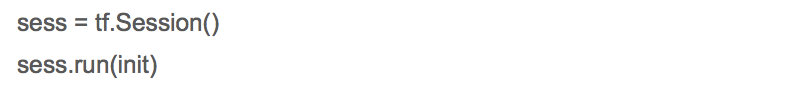Similarly we execute the train_step above within a loop by calling it withinsess.run().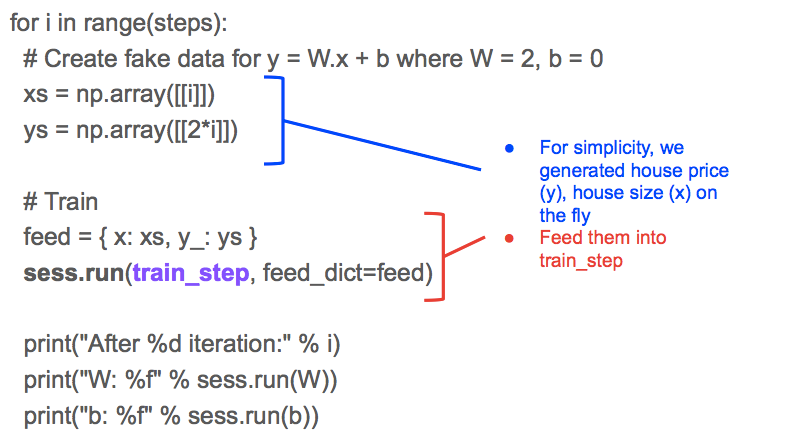The reason why you need to feed actual data points into feed, which is composed of x, y_ is that TF resolves the train_step into its dependencies: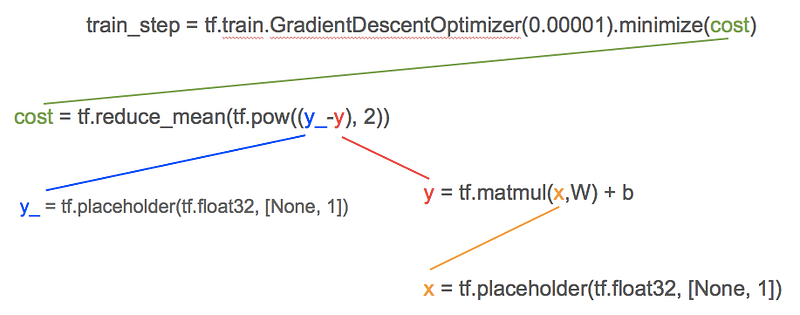At the bottom of the dependencies are the placeholders x, y_; and as we learned earlier tf.placeholders are used to indicate where we will feed actual data point values house price (y_), and house size (x).

Result

The print statement in the loop will show how TF learn the ‘good’ values for W, and b over each iteration.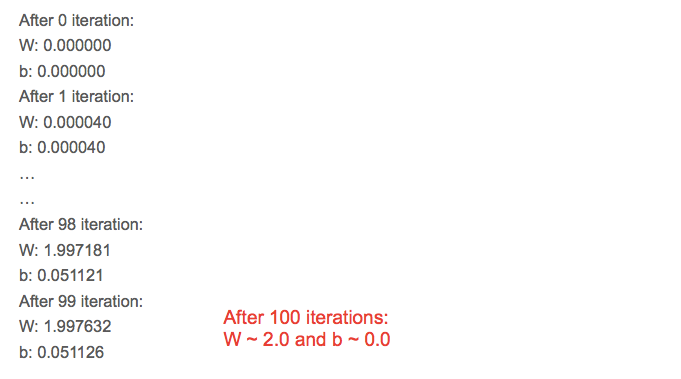### Wrapping Up

We have learned about Machine Learning in its simplest form; predict an outcome from a single feature. We chose a linear model (for simplicity) to fit our data points, define a cost function to represent best-fit, and train our model by repeatedly tweaking its gradient variable, W, and position variable b to minimize the cost function.

Coming Up Next

In the next article, we will:

• Set up Tensor Board to visualize TF execution to detect problems in our model, cost function, or gradient descent
• Feed data points in batches into the model during each training step (instead of just one data point at a time) to understand how it affects training

Resources

Bio: Soon Hin Khor, Ph.D is using tech to make the world more caring, and responsible. Contributor of ruby-tensorflow. Co-organizer for Tokyo Tensorflow meetup.

Original. Reposted with permission.

Related:

## Top Stories Past 30 Days

Most Popular
Most SharedGet KDnuggets, a leading newsletter on AI, Data Science, and Machine Learning